﻿ 列尾压强对列车纵向冲动影响研究

# 列尾压强对列车纵向冲动影响研究Research on the Influence of Train Pipe Tail Pressure on Train Longitudinal Impulse

Abstract: Heavy haul train is the development direction of railway freight transportation. With three acci-dents in 2020, the safety of heavy haul trains has attracted people’s attention again. All accidents of heavy haul trains originate from large coupler force, and the coupler force is the top priority when releasing. Due to the different release air charging time of heavy haul trains, there is usually a large difference in the pressure of train tail during re-braking, which has an impact on the train coupler force. In order to investigate the longitudinal impulse caused by the brake and release of trains with different pressure of train tail, this paper uses the Train Air Brake and Longitudinal Dynamics Simulation System to predict the brake and release of 20,000 t heavy haul trains under different pressure of train tail, and analyzes the level and causes of the longitudinal impulse generated in the process. The results show that the air brake force of the train with a lower pressure of train tail is weaker, the braking synchronization is poor, but the releasing synchronization is better. The train with a lower pressure of train tail will produce a larger compressing coupler force during air braking, but the tensile coupler force during the release will be greatly reduced. The train with high pressure of train tail is on the contrary.

1. 引言

2. 列车纵向动力学及空气制动系统

2.1. 列车纵向动力学模型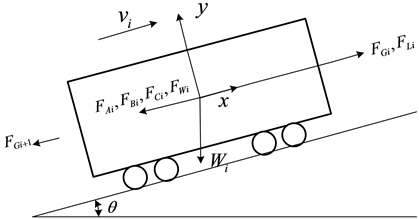Figure 1. The force diagram of a single vehicle

${F}_{1i}={F}_{Gi}-{F}_{Gi+1}+{F}_{L}{}_{i}-{F}_{Wi}$ (1)

${F}_{2i}=\left\{\begin{array}{ll}-\frac{{v}_{i}}{|{v}_{i}|}\left({F}_{Ai}+{F}_{Bi}\text{+}{F}_{Ci}\right)\hfill & {v}_{i}>0\hfill \\ -\left({F}_{Ai}+{F}_{Bi}\text{+}{F}_{Ci}\right)\hfill & {v}_{i}=0\text{\hspace{0.17em}}\text{and}\text{\hspace{0.17em}}{F}_{1i}\ge \left({F}_{Ai}+{F}_{Bi}\text{+}{F}_{Ci}\right)\hfill \\ -{F}_{1i}\hfill & {v}_{i}=0\text{\hspace{0.17em}}\text{and}\text{\hspace{0.17em}}{F}_{1i}<\left({F}_{Ai}+{F}_{Bi}\text{+}{F}_{Ci}\right)\hfill \\ 0\hfill & {v}_{i}=0\text{and}{F}_{1i}=\text{0}\hfill \end{array}$ (2)

${m}_{i}{\stackrel{¨}{x}}_{i}={F}_{1}{}_{i}+{F}_{2i}$ (3)

2.2. 列车空气制动系统模型

$\left\{\begin{array}{l}\frac{\partial \rho }{\partial t}+\rho \frac{\partial u}{\partial x}+u\frac{\partial \rho }{\partial x}+\frac{\rho u}{F}\frac{\text{d}F}{\text{d}x}={0}_{\begin{array}{l}\\ \end{array}}\hfill \\ \frac{\partial u}{\partial t}+u\frac{\partial u}{\partial x}+\frac{1}{\rho }\frac{\partial p}{\partial x}+\frac{4f}{D}\frac{{u}^{2}}{2}\frac{u}{|u|}=0\hfill \\ \frac{\partial p}{\partial t}+u\frac{\partial p}{\partial x}-{a}^{2}\frac{\partial \rho }{\partial t}-{a}^{2}u\frac{\partial \rho }{\partial x}-\left(k-1\right)\rho \left(q+u\frac{4f}{D}\frac{{u}^{2}}{2}\frac{u}{|u|}\right)=0\hfill \end{array}$ (4)

3. 列车管压强分布对制动特性的影响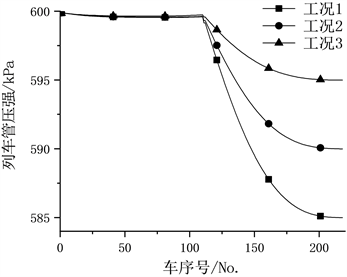Figure 2. Curve of the pressure of the train pipe under three working conditions at the initial timeTable 1. The average value of the brake cylinder balance pressure of the front and rear vehicles under three working conditionsTable 2. Comparison of train braking capacity under three working conditions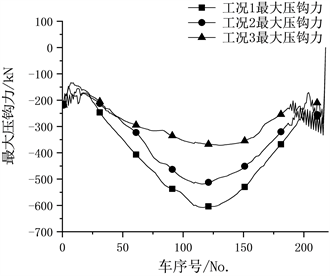Figure 3. Distribution of maximum compressing coupler force along length under three working conditions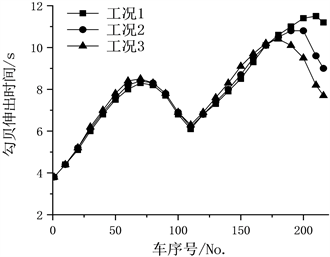Figure 4. Curve of the Train braking characteristic under three working conditions

4. 列车管压强分布对缓解特性的影响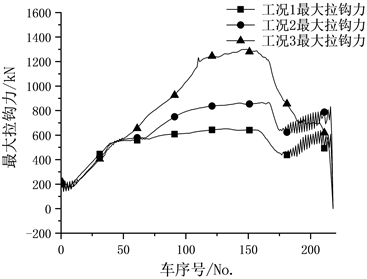Figure 5. Distribution of maximum tensile coupler forcealong length under three working conditions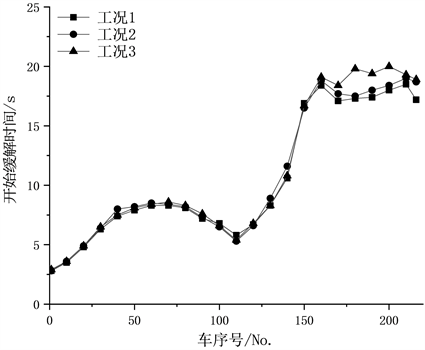Figure 6. Curve: Train release characteristic under three working conditions

5. 结论

1) 制动前列车的列车管压强分布影响制动后车钩力，列车管前后压强梯度越大，制动时车钩力越大。在减压50 kPa制动时，列尾压强为590 kPa、595 kPa的列车与585 kPa的列车相比，最大压钩力分别减小14.7%与38.9%。

2) 制动前列车管压强分布同样影响缓解后车钩力，列车管前后压强梯度越大，缓解时车钩力越小。在减压50 kPa制动并缓解时，列尾压强为590 kPa、595 kPa的列车相较列尾压强为585 kPa的列车最大拉钩力分别增加33.0%与99.4%。

3) 列车管压强分布影响制动时车钩力的原因主要是制动开始时间和后部车辆制动能力，列车后部车辆初始压强越低，制动时间越晚，制动力越弱，造成较大的前涌压缩车钩力。

4) 列车管压强分布影响缓解时车钩力的原因除缓解开始时间外同样是后部车辆制动能力，列车后部车辆初始压强越高，缓解时间越晚，制动力越强，造成较大的拉伸车钩力。

 Gruber, P. and Bayoumi, M.M. (1982) Suboptimal Control Strategies for Multilocomotive Powered Trains. IEEE Transac-tions on Automatic Control, 27, 536-546.
https://doi.org/10.1109/TAC.1982.1102986

 Cole, C. and Sun Y.Q. (2006) Simulated Comparisons of Wagon Coupler Systems in Heavy Haul Trains. Proceedings of the Institution of Mechanical Engineers, Part F: Journal of Rail and Rapid Transit, 220, 247-256.
https://doi.org/10.1243/09544097JRRT35

 Janarthanan, B., Padmanabhan, C. and Sujatha, C. (2012) Longitudinal Dynamics of a Tracked Vehicle: Simulation and Experiment. Journal of Terramechanics, 49, 63-72.
https://doi.org/10.1016/j.jterra.2011.11.001

 Spiryagin, M., Persson, I., Wu, Q., et al. (2019) A Co-Simulation Approach for Heavy Haul Long Distance Locomotive-Track Simulation Studies. Vehicle System Dynamics, 57, 1-18.
https://doi.org/10.1080/00423114.2018.1504088

 Eckert, J.J., Teodoro, C.P., Teixeira, L.H., et al. (2021) A Fast Simulation Approach to Assess Draft Gear Loads for Heavy Haul Trains During Braking. Mechanics Based Design of Structures and Machines.
https://doi.org/10.1080/15397734.2021.1875233

 李显洲. 列车纵向动力学模型研究及小间隙车钩动力学性能分析[D]: [硕士学位论文]. 大连: 大连铁道学院, 2002.

 魏伟, 赵旭宝, 姜岩, 等. 列车空气制动与纵向动力学集成仿真[J]. 铁道学报, 2012, 34(4): 39-46.

 Wu, Q., Spiryagin, M., Cole, C., et al. (2018) International Benchmarking of Longitudinal Train Dynamics Simulators: Results. Vehicle System Dynamics, 56, 343-365.
https://doi.org/10.1080/00423114.2017.1377840

 宋健, 魏伟. 重载列车纵向动力学仿真模型的有效性研究[J]. 大连交通大学学报, 2019, 40(3): 23-29.

 魏伟, 李文辉. 列车空气制动系统数值仿真[J]. 铁道学报, 2003, 25(1): 38-42.

 魏伟. 列车空气制动系统仿真的有效性[J]. 中国铁道科学, 2006, 27(5): 104-109.

Top